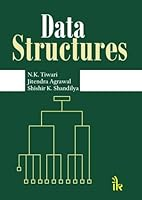# Data Structures## Book Description

Data structure is a way of organizing data in a computer memory so that it can be used efficiently. Data structures can implement one or more particular abstract data types (ADT), which are the means of specifying the nature of operations and their complexity. This book focuses on the design and analysis of basic data structures and their implementation and intends to provide a strong conceptual and empirical understanding of data structures. The contents of this book will help the students to understand that how the data structures are implemented and why these implementations are vital. The initial chapters deal with introductory part of the subject and the later chapters focus on advanced and difficult problems and algorithms. The book explains how data is organized in computer memory; how it operates; and why it is important. Appendixes provide further information on algorithms along with their programming solutions. Readers of this book need only to be familiar with the basic syntax of C/C++ and similar languages. This book will be useful for B.Tech/M.Tech Computer Science and Engineering, IT Engineering, BSc (IT)/MSc (IT), and BCA/MCA students.

Chapter 1. Introduction
Chapter 2. Array
Chapter 3. Recursion
Chapter 4. Stack
Chapter 5. Queue
Chapter 6. List
Chapter 7. Tree
Chapter 8. Graph Theory
Chapter 9. Sorting and Searching
Chapter 10. Tables